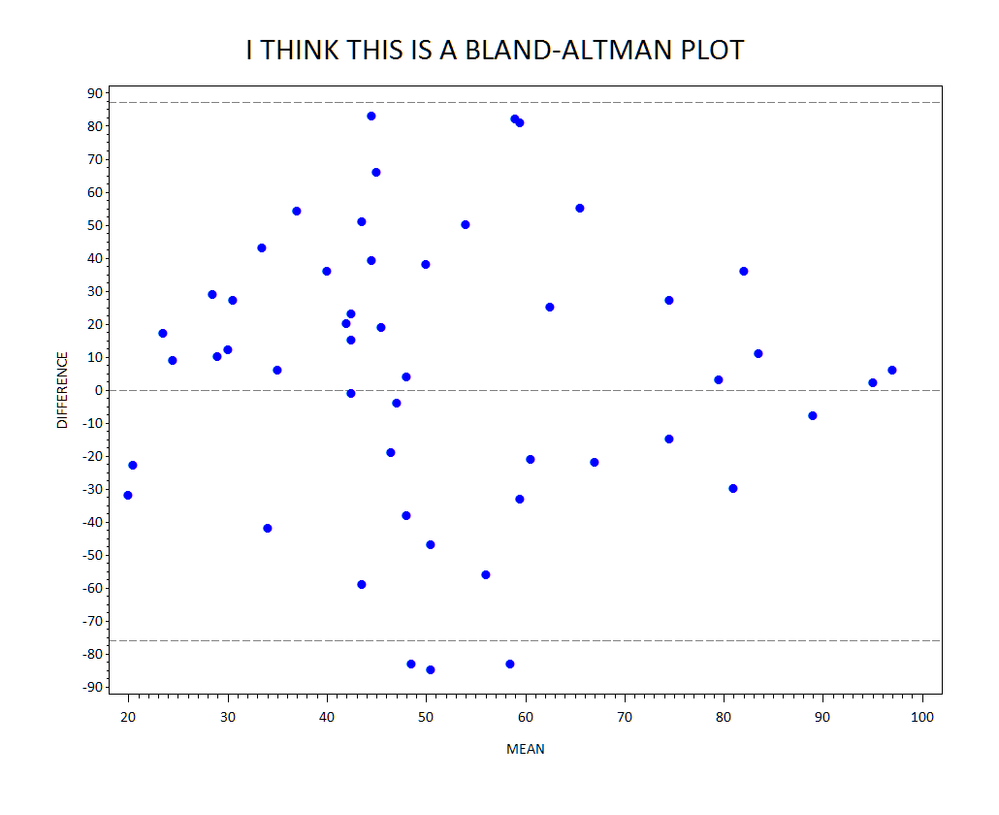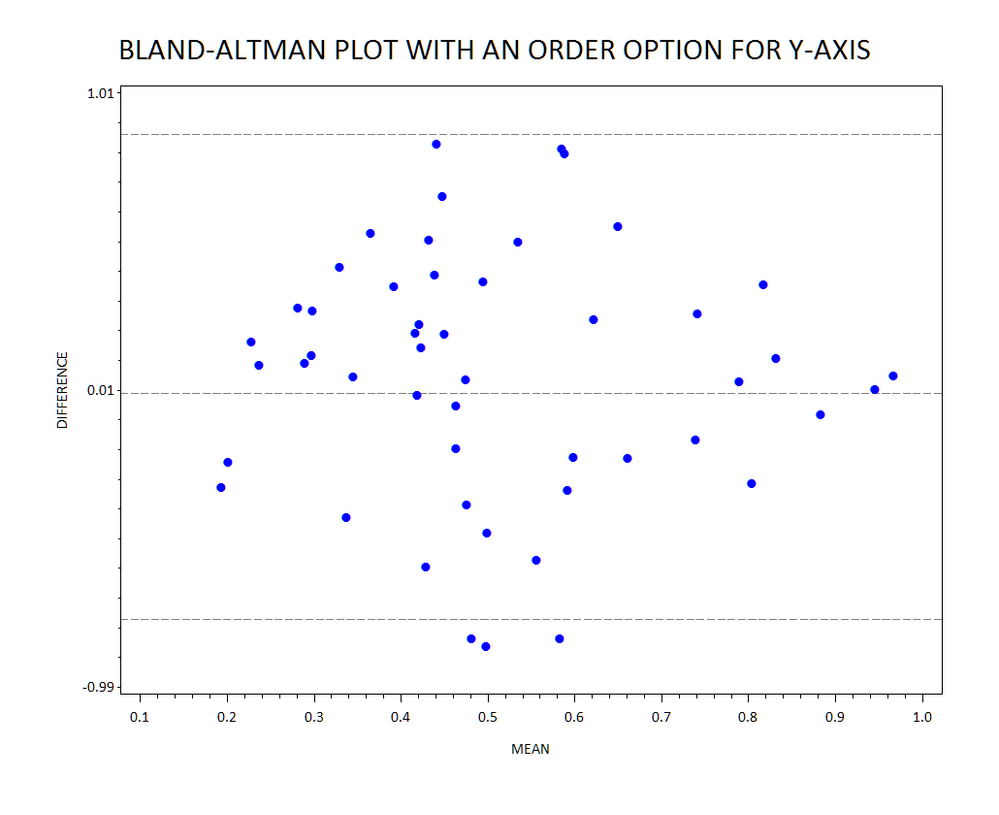## example of creating Bland-altman plots

Hi All,

Would someone please provide examples to creating Bland-altman
plots ?

Thanks!

1 ACCEPTED SOLUTION

Accepted SolutionsMikeZdeb
Rhodochrosite | Level 12

## Re: example of creating Bland-altman plots

hi ... from what I know about Bland-Altman plots ...

* some fake data;

data test;

do _n_ = 1 to 50;

m1 = ceil(100*ranuni(999));

m2 = ceil(100*ranuni(999));

output;

end;

run;

* compute means (x), difference (y);

data bla_alt;

set test;

x = mean(of m:);

y = m1 - m2;

run;

* compute mean +/- 1.96 standard deviation to add reference lines to the plot;

proc sql;

select mean(y)-1.96*std(y), mean(y)+1.96*std(y) into :lo, :hi from bla_alt;

quit;

* reset graphics options;

goptions reset=all ftext='calibri' htext=2 gunit=pct;

title1 h=4 'I THINK THIS IS A BLAND-ALTMAN PLOT' ls=2;

* whitespace around plot (if desired);

title2 a=90 ls=2;

title3 a=-90 ls=2;

footnote1 ls=1;

* a blue dot for the symbol;

symbol1 f='wingdings' v='6c'x c=blue h=2;

axis1 offset=(2,2)pct label=('MEAN');

axis2 label=(a=90 'DIFFERENCE');

* plot with dashed reference lines;

proc gplot bla_alt;

plot y*x / haxis=axis1 vaxis=axis2 vref=&lo,0,&hi lvref=3;

run;

quit;

ps attached plot looks like ... Bland–Altman plot - Wikipedia, the free encyclopedia4 REPLIES 4MikeZdeb
Rhodochrosite | Level 12

## Re: example of creating Bland-altman plots

hi ... from what I know about Bland-Altman plots ...

* some fake data;

data test;

do _n_ = 1 to 50;

m1 = ceil(100*ranuni(999));

m2 = ceil(100*ranuni(999));

output;

end;

run;

* compute means (x), difference (y);

data bla_alt;

set test;

x = mean(of m:);

y = m1 - m2;

run;

* compute mean +/- 1.96 standard deviation to add reference lines to the plot;

proc sql;

select mean(y)-1.96*std(y), mean(y)+1.96*std(y) into :lo, :hi from bla_alt;

quit;

* reset graphics options;

goptions reset=all ftext='calibri' htext=2 gunit=pct;

title1 h=4 'I THINK THIS IS A BLAND-ALTMAN PLOT' ls=2;

* whitespace around plot (if desired);

title2 a=90 ls=2;

title3 a=-90 ls=2;

footnote1 ls=1;

* a blue dot for the symbol;

symbol1 f='wingdings' v='6c'x c=blue h=2;

axis1 offset=(2,2)pct label=('MEAN');

axis2 label=(a=90 'DIFFERENCE');

* plot with dashed reference lines;

proc gplot bla_alt;

plot y*x / haxis=axis1 vaxis=axis2 vref=&lo,0,&hi lvref=3;

run;

quit;

ps attached plot looks like ... Bland–Altman plot - Wikipedia, the free encyclopedia## Re: example of creating Bland-altman plots

Hi Mike,

Thank you very much for your help!  When I use my real data I got a warning:

WARNING: The reference line at 0.134745 on the vertical axis labeled __lvedd_diff is out of range.

How should I modify your code?MikeZdeb
Rhodochrosite | Level 12

## Re: example of creating Bland-altman plots

hi ... GPLOT chooses the min and max for the Y-axis based on a look at your data values

the calculated value for the reference line must be outside the values chosen for the bounds of the y-axis

you can use an ORDER option on an AXIS statement to control the min and max

here's an example with measurement values between 0 and 1 where the min and max on the y-axis will always be outside the ref lines

the level of ROUNDING in PROC SQL would have to be dictated by the range of your data values (I chose round to hundreths but that might not always be apppropriate)

data test;

do _n_ = 1 to 50;

m1 = ranuni(999);

m2 = ranuni(999);

output;

end;

run;

data bla_alt;

set test;

x = mean(of m:);

y = m1 - m2;

run;

proc sql noprint;

select mean(y)-1.96*std(y), mean(y)+1.96*std(y),

round(mean(y)-2.5*std(y),0.01), round(mean(y)+2.5*std(y),0.01)

into :lo, :hi , :min , :max from bla_alt;

quit;

goptions reset=all ftext='calibri' htext=2 gunit=pct;

title1 h=4 'BLAND-ALTMAN PLOT WITH AN ORDER OPTION FOR Y-AXIS' ls=2;

* whitespace;

title2 a=90 ls=2;

title3 a=-90 ls=2;

footnote1 ls=1;

symbol1 f='wingdings' v='6c'x c=blue h=2;

axis1 offset=(2,2)pct label=('MEAN');

* min and max macro variables used for range of y-axis;

axis2 label=(a=90 'DIFFERENCE') order=&min to &max;

proc gplot data=bla_alt;

plot y*x / haxis=axis1 vaxis=axis2 vref=&lo,0,&hi lvref=3;

run;

quit;## Re: example of creating Bland-altman plots

Hi Mike,

It works great! Thank you very much!!!

Discussion stats
• 4 replies
• 5066 views
• 3 likes
• 2 in conversation